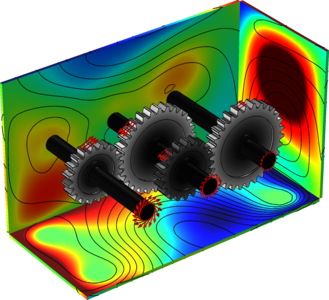# Galleria delle Applicazioni

## Vibrations in a Compound Gear Train

Application ID: 36291

This model simulates vibrations in a compound gear train. Spur gears, used to model the gear train, are mounted on rigid shafts. The shafts are supported by an elastic housing at both ends. The gear mesh is assumed to be elastic with varying stiffness, which is the source of vibration. A transient analysis is performed to compute the dynamics of the gears as well as the vibrations in the housing.

Contact modeling is used for the computation of the gear mesh stiffness. A parametric analysis is performed to compute the gear mesh stiffness as a function of gear rotation in one mesh cycle.

The model calculates the von Mises stress distribution in the gear pair, where the stresses are high at the contact points, as well as at the roots of the teeth. The gear mesh stiffness, displacement in the gears, and the normal acceleration in the housing due to vibration are also calculated.This model example illustrates applications of this type that would nominally be built using the following products: